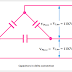Most of the time capacitor bank is connected in Delta, but it also connected in Star for some applications. Now the question is arise when Capacitor bank is connected in Star and when connected in Delta? Which connection is better for Capacitor Bank Star or Delta? In this article, you will find the advantages and disadvantages of both star connected capacitor bank and delta connected capacitor bank.

The capacitor bank is used for power factor correction. For the correction of the power factor in the three-phase system, three phase capacitor bank is needed which may be star connected or delta connected.

## Delta connected Capacitor Bank

Delta connected capacitor banks are generally used for low to medium voltage. The delta connected capacitor bank can be used for high voltage but sometimes it is not possible because in delta connection the full phase voltage applied across each capacitor whereas in star connection root 3 times lesser than phase voltage is applied across the capacitor.

So you may understand if we use delta connected capacitor bank at high voltage, the voltage rating of the capacitor should be high. So high voltage capacitor manufacturing is costly and sometimes impossible.

### Advantages of Delta Connection in a Capacitor Bank

1. The KVAR generated by the capacitor is proportional to the square of the applied voltage that means if the voltage is more the KVAR also be more. So the delta connected capacitor bank provides more KVAR compared to the star connected capacitor bank because in star connection less voltage applied across the capacitor than delta connection.

2. Delta connected Capacitor bank can circulate the harmonic current, so it can reduce the harmonic effect in an electrical system.

3. Delta connected capacitor bank provides a balanced capacitance to each phase of the electrical system and also maintains a balanced voltage.

4. If a capacitor cell in one phase is failed inside the capacitor bank, the voltage across each phase remains the same, only KVAR is dropped.

### Disadvantage of Delta Connection in a Capacitor Bank

1. The only disadvantage of the delta connected capacitor bank is, the voltage stress across each capacitor is high which reduce the life of the capacitor and it may not be used for high voltage applications.

## Star connected Capacitor Bank

The star connected capacitor bank is used for medium to high voltage applications. In star connection, the voltage across each capacitor is root 3 times lesser than the phase voltage, so the voltage stress across the capacitors are low even in high voltage applications. There are two types of star connection in the capacitor bank.
1. Grounded Star Connection
2. Ungrounded star Connection

In grounded star connection, the neutral point is connected to earth or it is grounded but in ungrounded star connection, the neutral point is isolated from the earth or ground.

### Advantages of Star Connected Capacitor Bank

1. The star connected capacitor bank simple in connection.
2. The voltage stress across each capacitor is less, so the life of the capacitors are high.

### Disadvantages of Star Connected Capacitor Bank

1. Star connected capacitor bank provides less KVAR than delta connected capacitor bank because the voltage across the capacitor is less.

2. Star connected capacitor bank cannot circulate the harmonic current in an electrical system.

3. The ungrounded star connected capacitor bank cannot maintain the balance voltage and cannot provide the balance capacitance.

4. If a capacitor cell in one phase is failed, the unbalanced voltage occurs in the electrical system.

Conclusion

You may understand the delta connected capacitor bank provides more advantages and benefits than the star connected capacitor bank. So delta connection is better for capacitor bank. For these reasons, capacitor banks are mostly delta connected.

Which connection is better for Capacitor Bank Star or Delta?Reviewed by manoranjan das on 7/01/2019 Rating: 5